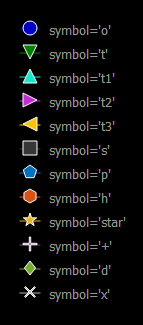Related Articles
PyQtGraph – Symbols
• Last Updated : 01 Nov, 2020

PyQtGraph is a graphics and user interface Python library for functionalities commonly required in designing and science applications. Its provides fast, interactive graphics for displaying data (plots, video, etc.). A line chart is a type of graph which displays information as a series of data points commonly known as markers, connected by straight line segments. A scatter plot uses dots to represent values for two different numeric variables. It is a type of graph, drawn using Cartesian coordinates to display values for typically two variables for a set of data

When we create a line graph or scatter plot graph, the points which represent some data needs to be highlighted using certain symbols, by default it is round circle but this can be modified as required. There are a total of 12 symbols which are offered by pyqtgraph which can be used to change symbol as required.
Below are the list of the symbols available in this module:

• o : Default symbol, round circle symbol
• t : Triangle pointing downwards symbol
• t1 : Triangle pointing upwards symbol
• t2 : Triangle pointing right side symbol
• t3 : Triangle pointing left side symbol
• s : Square symbol
• p : Pentagon symbol
• h : Hexagon symbol
• star : Star symbol
• + : Plus symbol
• d : Prism symbol
• x : Cross symbol

This is how they appear on the output screen:We can create a plot window with the help of commands given below :

```plt = pg.plot()
```

In order to implement the above explained concept, following steps can be followed:

•  Import pyqtgraph, pyqt5 and numpy modules
• Create Main window class
• Create a plot window object
• Add legend to the plot window
• Create 12 lines having different points so that they don’t intersect each other and hiving different symbol and colors
• Add the plot window and other widget like label to the grid layout
• Set grid layout widget as the central widget of the main window

Example:

## Python3

 `# importing Qt widgets``from` `PyQt5.QtWidgets ``import` `*`` ` `# importing system``import` `sys`` ` `# importing numpy as np``import` `numpy as np`` ` `# importing pyqtgraph as pg``import` `pyqtgraph as pg``from` `PyQt5.QtGui ``import` `*` `from` `PyQt5.QtCore ``import` `*` `from` `collections ``import` `namedtuple`` ` ` ` `class` `Window(QMainWindow):`` ` `    ``def` `__init__(``self``):``        ``super``().__init__()`` ` `        ``# setting title``        ``self``.setWindowTitle(``"PyQtGraph"``)`` ` `        ``# setting geometry``        ``self``.setGeometry(``100``, ``100``, ``800``, ``500``)`` ` ` ` `        ``# icon``        ``icon ``=` `QIcon(``"skin.png"``)`` ` `        ``# setting icon to the window``        ``self``.setWindowIcon(icon)`` ` `        ``# calling method``        ``self``.UiComponents()`` ` `        ``# showing all the widgets``        ``self``.show()`` ` `    ``# method for components``    ``def` `UiComponents(``self``):`` ` `        ``# creating a widget object``        ``widget ``=` `QWidget()`` ` `        ``# text``        ``text ``=` `"Geeksforgeeks Different Symbols"`` ` `        ``# creating a label``        ``label ``=` `QLabel(text)`` ` `        ``# setting minimum width``        ``label.setMinimumWidth(``130``)`` ` `        ``# making label do word wrap``        ``label.setWordWrap(``True``)`` ` `        ``# setting pyqtgraph configuration``        ``pg.setConfigOptions(antialias ``=` `True``)`` ` `        ``# creating a plot window``        ``plot ``=` `pg.plot()`` ` `        ``# adding legend to the plot window``        ``plot.addLegend()`` ` `        ``# plot the line1 with symbol o``        ``# having color blue``        ``line1 ``=` `plot.plot([``1``, ``1``, ``1``, ``1``, ``1``], pen ``=``(``0``, ``0``, ``200``), symbolBrush ``=``(``0``, ``0``, ``200``),``                          ``symbolPen ``=``'w'``, symbol ``=``'o'``, symbolSize ``=` `14``, name ``=``"symbol ='o'"``)`` ` `        ``# plot the line2 with symbol t``        ``# having color green``        ``line2 ``=` `plot.plot([``2``, ``2``, ``2``, ``2``, ``2``], pen ``=``(``0``, ``128``, ``0``), symbolBrush ``=``(``0``, ``128``, ``0``),``                          ``symbolPen ``=``'w'``, symbol ``=``'t'``, symbolSize ``=` `14``, name ``=``"symbol ='t'"``)`` ` `        ``# plot the line3 with symbol t1``        ``# having color bluegreen``        ``line3 ``=` `plot.plot([``3``, ``3``, ``3``, ``3``, ``3``], pen ``=``(``19``, ``234``, ``201``), symbolBrush ``=``(``19``, ``234``, ``201``),``                          ``symbolPen ``=``'w'``, symbol ``=``'t1'``, symbolSize ``=` `14``, name ``=``"symbol ='t1'"``)`` ` `        ``# plot the line4 with symbol t2``        ``# having color purple``        ``line4 ``=` `plot.plot([``4``, ``4``, ``4``, ``4``, ``4``], pen ``=``(``195``, ``46``, ``212``), symbolBrush ``=``(``195``, ``46``, ``212``),``                          ``symbolPen ``=``'w'``, symbol ``=``'t2'``, symbolSize ``=` `14``, name ``=``"symbol ='t2'"``)`` ` `        ``# plot the line5 with symbol t3``        ``# having color brownish``        ``line5 ``=` `plot.plot([``5``, ``5``, ``5``, ``5``, ``5``], pen ``=``(``250``, ``194``, ``5``), symbolBrush ``=``(``250``, ``194``, ``5``),``                          ``symbolPen ``=``'w'``, symbol ``=``'t3'``, symbolSize ``=` `14``, name ``=``"symbol ='t3'"``)`` ` `        ``# plot the line6 with symbol s``        ``# having color grey``        ``line6 ``=` `plot.plot([``6``, ``6``, ``6``, ``6``, ``6``], pen ``=``(``54``, ``55``, ``55``), symbolBrush ``=``(``55``, ``55``, ``55``), symbolPen ``=``'w'``, symbol ``=``'s'``, symbolSize ``=` `14``,``                  ``name ``=``"symbol ='s'"``)`` ` `        ``# plot the line7 with symbol p``        ``# having color sky blue``        ``line7 ``=` `plot.plot([``7``, ``7``, ``7``, ``7``, ``7``], pen ``=``(``0``, ``114``, ``189``), symbolBrush ``=``(``0``, ``114``, ``189``),``                         ``symbolPen ``=``'w'``, symbol ``=``'p'``, symbolSize ``=` `14``, name ``=``"symbol ='p'"``)`` ` `        ``# plot the line8 with symbol h``        ``# having color orange``        ``line8 ``=` `plot.plot([``8``, ``8``, ``8``, ``8``, ``8``], pen ``=``(``217``, ``83``, ``25``), symbolBrush ``=``(``217``, ``83``, ``25``),``                          ``symbolPen ``=``'w'``, symbol ``=``'h'``, symbolSize ``=` `14``, name ``=``"symbol ='h'"``)`` ` `        ``# plot the line9 with symbol star``        ``# having yellow color``        ``line9 ``=` `plot.plot([``9``, ``9``, ``9``, ``9``, ``9``], pen ``=``(``237``, ``177``, ``32``), symbolBrush ``=``(``237``, ``177``, ``32``),``                          ``symbolPen ``=``'w'``, symbol ``=``'star'``, symbolSize ``=` `14``, name ``=``"symbol ='star'"``)`` ` `        ``# plot the line10 with symbol +``        ``# having blueish color``        ``line10 ``=` `plot.plot([``10``, ``10``, ``10``, ``10``, ``10``], pen ``=``(``126``, ``47``, ``142``), symbolBrush ``=``(``126``, ``47``, ``142``),``                           ``symbolPen ``=``'w'``, symbol ``=``'+'``, symbolSize ``=` `14``, name ``=``"symbol ='+'"``)`` ` `        ``# plot the line11 with symbol d``        ``# having greensih color``        ``line11 ``=` `plot.plot([``11``, ``11``, ``11``, ``11``, ``11``], pen ``=``(``119``, ``172``, ``48``), symbolBrush ``=``(``119``, ``172``, ``48``),``                           ``symbolPen ``=``'w'``, symbol ``=``'d'``, symbolSize ``=` `14``, name ``=``"symbol ='d'"``)`` ` `        ``# plot the line12 with symbol x``        ``# having off white color``        ``line12 ``=` `plot.plot([``12``, ``12``, ``12``, ``12``, ``12``], pen ``=``(``180``, ``180``, ``180``), symbolBrush ``=``(``180``, ``180``, ``180``),``                           ``symbolPen ``=``'w'``, symbol ``=``'x'``, symbolSize ``=` `14``, name ``=``"symbol ='x'"``)`` ` `        ``# setting x-axis range``        ``plot.setXRange(``-``3``, ``4``)`` ` `        ``# Creating a grid layout``        ``layout ``=` `QGridLayout()`` ` `        ``# minimum width value of the label``        ``label.setMinimumWidth(``130``)`` ` `        ``# setting this layout to the widget``        ``widget.setLayout(layout)`` ` `        ``# adding label in the layout``        ``layout.addWidget(label, ``1``, ``0``)`` ` `        ``# plot window goes on right side, spanning 3 rows``        ``layout.addWidget(plot, ``0``, ``1``, ``3``, ``1``)`` ` `        ``# setting this widget as central widget of the main widow``        ``self``.setCentralWidget(widget)`` ` ` ` ` ` `# create pyqt5 app``App ``=` `QApplication(sys.argv)`` ` `# create the instance of our Window``window ``=` `Window()`` ` `# start the app``sys.exit(App.``exec``())`

Output :

Attention geek! Strengthen your foundations with the Python Programming Foundation Course and learn the basics.

To begin with, your interview preparations Enhance your Data Structures concepts with the Python DS Course. And to begin with your Machine Learning Journey, join the Machine Learning – Basic Level Course

My Personal Notes arrow_drop_up